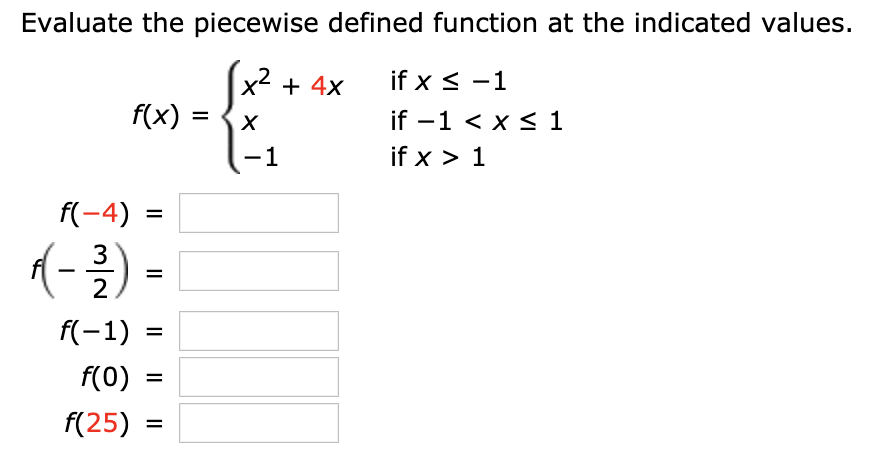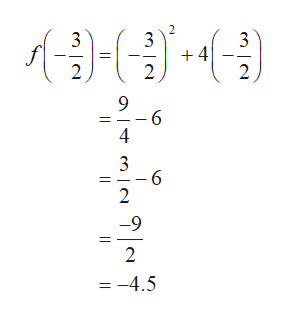# Evaluate the piecewise defined function at the indicated values.x2f(x)if x -1+ 4xif -1 < x s 1Xif x 11f(-4)1-32f(-1)f(0)=f(25)=II

Questionhelp_outlineImage TranscriptioncloseEvaluate the piecewise defined function at the indicated values. x2 f(x) if x -1 + 4x if -1 < x s 1 X if x 1 1 f(-4) 1- 3 2 f(-1) f(0) = f(25) = II fullscreen
check_circleExpert Solution
Step 1

Substitute the value x=-4 in f(x)=x^2+4x.

Step 2

Substitute the value x=-3/2 in f(x)=x^2+4x.help_outlineImage Transcriptionclose2 3 +4 2 3 3 2 2 9 - 6 4 3 2 9 2 = -4.5 fullscreen
Step 3

Substitute the value x=-1 in f(x)=x^2+...

### Want to see the full answer?

See Solution

#### Want to see this answer and more?

Solutions are written by subject experts who are available 24/7. Questions are typically answered within 1 hour*

See Solution
*Response times may vary by subject and question
Tagged in

### Other